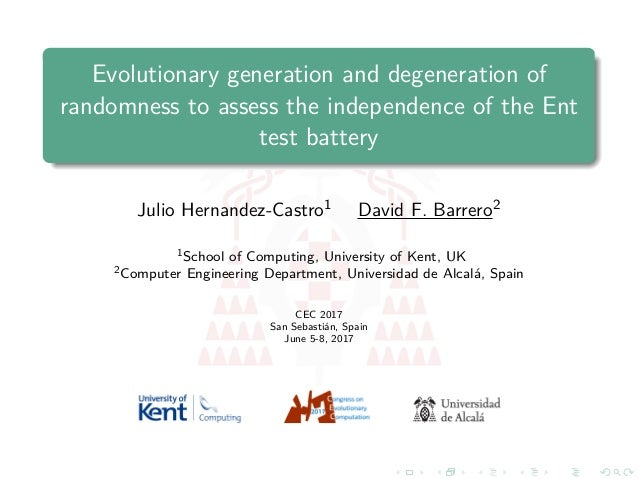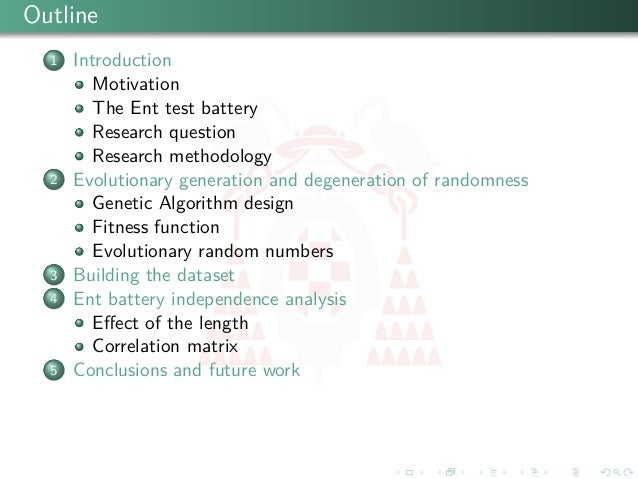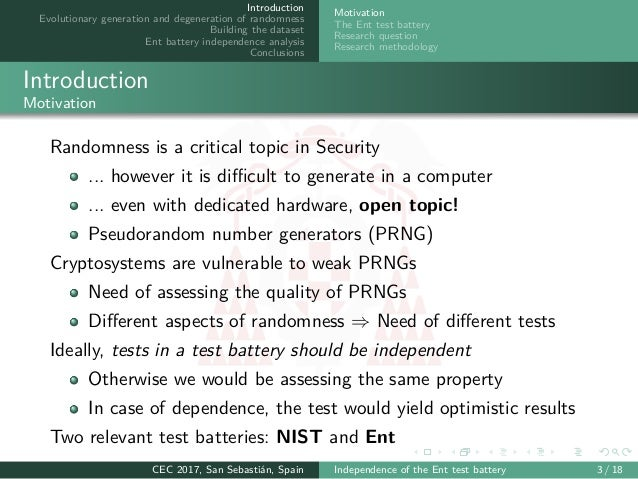Successfully reported this slideshow.Upcoming SlideShare
×

of0

Share

# Evolutionary generation and degeneration of randomness to assess the independence of the Ent test battery

Randomness tests are a key tool to assess the quality of pseudo-random and true random (physical) number generators. They exploit some properties of random numbers to quantify to which extent the observed behavior of the tested sequence approximates the expected one. Given the many sides of randomness, there is not an unique test providing the whole picture, it is needed instead a suite of tests assessing different aspects randomness. A robust test suite must include independent tests, otherwise tests would assess the same property, providing redundant information. This paper addresses the independence assessment of a popular test suite named Ent. To this end we generate a large number of pseudo-random numbers with different degrees of randomness by evolving them with a Genetic Algorithm. The numbers are generated to maximize their diversity attending different criteria based on Ent output, used as fitness. We encourage diversity by maximizing and minimizing randomness measures. Once a diverse set of pseudo-random numbers is generated, the Ent test suite is run on them, and their statistics studied by means of a classical correlation analysis. The results show high correlation among some statistics used in the literature, which could be overestimating the quality of their randomness source.

### Related Books

#### Free with a 30 day trial from Scribd

See all
• Be the first to like this

### Evolutionary generation and degeneration of randomness to assess the independence of the Ent test battery

1. 1. Evolutionary generation and degeneration of randomness to assess the independence of the Ent test battery Julio Hernandez-Castro1 David F. Barrero2 1School of Computing, University of Kent, UK 2Computer Engineering Department, Universidad de Alcalá, Spain CEC 2017 San Sebastián, Spain June 5-8, 2017
2. 2. Outline 1 Introduction Motivation The Ent test battery Research question Research methodology 2 Evolutionary generation and degeneration of randomness Genetic Algorithm design Fitness function Evolutionary random numbers 3 Building the dataset 4 Ent battery independence analysis Eﬀect of the length Correlation matrix 5 Conclusions and future work
3. 3. Introduction Evolutionary generation and degeneration of randomness Building the dataset Ent battery independence analysis Conclusions Motivation The Ent test battery Research question Research methodology Introduction Motivation Randomness is a critical topic in Security ... however it is diﬃcult to generate in a computer ... even with dedicated hardware, open topic! Pseudorandom number generators (PRNG) Cryptosystems are vulnerable to weak PRNGs Need of assessing the quality of PRNGs Diﬀerent aspects of randomness ⇒ Need of diﬀerent tests Ideally, tests in a test battery should be independent Otherwise we would be assessing the same property In case of dependence, the test would yield optimistic results Two relevant test batteries: NIST and Ent CEC 2017, San Sebastián, Spain Independence of the Ent test battery 3 / 18
4. 4. Introduction Evolutionary generation and degeneration of randomness Building the dataset Ent battery independence analysis Conclusions Motivation The Ent test battery Research question Research methodology Introduction The Ent test battery (I) Ent is an open source randomness test battery implementation Compute a statistic and derive a p-value for a null hypothesis Ent contains ﬁve tests/statistics Entropy Entropy as deﬁned in Information Theory Chi-square (χ2) Compares expected versus observed frequencies Arithmetic mean Arithmetic mean of the symbols Monte-Carlo Pi Monte-Carlo simulation to estimate π. Measured as error of the estimation (pierror) Serial correlation Correlation between two consecutive symbols CEC 2017, San Sebastián, Spain Independence of the Ent test battery 4 / 18
5. 5. Introduction Evolutionary generation and degeneration of randomness Building the dataset Ent battery independence analysis Conclusions Motivation The Ent test battery Research question Research methodology Introduction The Ent test battery (II) Ent output example Entropy = 4.385614 bits per byte. Optimum compression would reduce the size of this 20180 byte file by 45 percent. Chi square distribution for 20180 samples is 1266758.61, and randomly would exceed this value less than 0.01 percent of the times. Arithmetic mean value of data bytes is 56.5619 (127.5 = random). Monte Carlo value for Pi is 3.611061552 (error 14.94 percent). Serial correlation coefficient is 0.568671 (totally uncorrelated = 0.0). CEC 2017, San Sebastián, Spain Independence of the Ent test battery 5 / 18
6. 6. Introduction Evolutionary generation and degeneration of randomness Building the dataset Ent battery independence analysis Conclusions Motivation The Ent test battery Research question Research methodology Introduction Research question Research question Are the Ent tests independent? Hard analytical approach ⇒ Experimental approach Generate random numbers, run the tests and apply statistical tools to ﬁnd dependencies The (scarce) literature on this topic uses p-values Good to compare tests and relate them to tests results Non-linear transformations that loose potentially valuable information We focus on the statistics CEC 2017, San Sebastián, Spain Independence of the Ent test battery 6 / 18
7. 7. Introduction Evolutionary generation and degeneration of randomness Building the dataset Ent battery independence analysis Conclusions Motivation The Ent test battery Research question Research methodology Introduction Research methodology Step I: Generate pseudorandom numbers Problem: Potential bias by the PRNG Solution: Use a GA Step II: Run the tests Store Ent statistics Step III: Study the independence Classical correlation analysis Generate numbers Run Ent Statistical analysis GA Store statistics Correlation study CEC 2017, San Sebastián, Spain Independence of the Ent test battery 7 / 18
8. 8. Introduction Evolutionary generation and degeneration of randomness Building the dataset Ent battery independence analysis Conclusions Genetic Algorithm design Fitness function Evolutionary random numbers Evolutionary generation and degeneration of randomness Genetic algorithm design Potential biases by PRNG Too weak (or strong) PRNG Solution: “Randomize” random numbers New problems Any randomization could induce new biases Solution: GA with diﬀerent ﬁtnesses Population 100 Codiﬁcation Binary ﬁxed size Length {2i }∀i = 7, . . . , 17 Initial pop Random, T-M PRNG Crossover One-point Mutation Bit ﬂip, pm = 0,005 Selection Tournament size two Elitism 1 CEC 2017, San Sebastián, Spain Independence of the Ent test battery 8 / 18
9. 9. Introduction Evolutionary generation and degeneration of randomness Building the dataset Ent battery independence analysis Conclusions Genetic Algorithm design Fitness function Evolutionary random numbers Evolutionary generation and degeneration of randomness Fitness function (I) The objective of the GA is to enhance randomness diversity No theoretical clues about how to include or remove randomness Lack of a general randomness theory Idea: Use Ent output as ﬁtness Seven Ent statistics: Entropy, compression, χ2, excess, average mean, pierror, serial correlation Statistics usually found in the literature GA run for maximization and minimization CEC 2017, San Sebastián, Spain Independence of the Ent test battery 9 / 18
10. 10. Introduction Evolutionary generation and degeneration of randomness Building the dataset Ent battery independence analysis Conclusions Genetic Algorithm design Fitness function Evolutionary random numbers Evolutionary generation and degeneration of randomness Fitness function (II) All ﬁtnessess were formulated as maximization Tournament selects the best or worse Ent statistic Fitness value Entropy fentropy = Entropy Compression fcompression = 100 − Compression Chisquared fchisquared = 1 1+Chisquared Excess fexcess = Excess Average mean famean = 1 (1+|127,5−amean|) Pierror fpierror = 1 1+pierror Correlation fcorrelation = 1 1+|correlation| CEC 2017, San Sebastián, Spain Independence of the Ent test battery 10 / 18
11. 11. Evolutionary generation and degeneration of randomness Evolutionary random numbers Maximization Generation Meanfitness 0.00400.00450.00500.0055 0 10 20 30 40 50 chisquared 5060708090100 0 10 20 30 40 50 compression 0.850.900.951.00 0 10 20 30 40 50 correlation 45678 0 10 20 30 40 50 entropy 5060708090100 0 10 20 30 40 50 excess 0.20.40.60.8 0 10 20 30 40 50 mean 0.00.20.40.6 0 10 20 30 40 50 pierror Length 128 256 512 1024 8192 16384 32768 Minimization Generation Meanfitness 0.00.10.20.30.40.5 0 10 20 30 40 50 pierror 0.00100.00150.00200.00250.00300.00350.0040 0 10 20 30 40 50 chisquared 406080100 0 10 20 30 40 50 compression 0.50.60.70.80.91.0 0 10 20 30 40 50 correlation 345678 0 10 20 30 40 50 entropy 01020304050 0 10 20 30 40 50 excess 0.00.10.20.30.40.50.6 0 10 20 30 40 50 mean Length 128 256 512 1024 8192 16384 32768
12. 12. Introduction Evolutionary generation and degeneration of randomness Building the dataset Ent battery independence analysis Conclusions Building the dataset GA populations were stored Direct dump of chromosomes to disk 1, 559, 880 numbers generated in minimization 1, 559, 880 numbers generated in maximization Ent statistics of each number were computed and stored Seven statistics per number Analysis in two phases 1 Eﬀect of the sequence length 2 Correlation analysis CEC 2017, San Sebastián, Spain Independence of the Ent test battery 12 / 18
13. 13. Ent battery independence analysis Eﬀect of the sequence length 1024 128 256 512 8192 entropy 0 10 30 50 90 110 130 150 0 20 60 100 45678 0103050 compression chisquared 200250300 90110130150 mean pierror 02060100 02060100 excess 4 5 6 7 8 200 250 300 0 20 60 100 −0.8 −0.2 0.2 0.6 −0.8−0.20.20.6 correlation
14. 14. Introduction Evolutionary generation and degeneration of randomness Building the dataset Ent battery independence analysis Conclusions Eﬀect of the length Correlation matrix Ent battery independence analysis Correlation analysis Correlation matrix with l = 8, 192 bits (maximization / minimization) Ent. Compression χ2 Mean Pierror Excess Correlation Ent. 1.0 -0.80 / -0.81 -0.98 / -0.98 0.11 / -0.12 0.06 / 0.07 0.90 / 0.83 -0.09 / -0.08 Comp. - 1.0 0.78 / 0.80 -0.11 / 0.09 -0.05 / -0.07 -0.62 / -0.52 0.09 / 0.06 χ2 - - 1.0 -0.11 / 0.11 -0.06 / -0.07 -0.92, -0.84 0.09 / 0.08 Mean - - - 1.0 -0.00 / 0.32 0.09 / -0.10 -0.01 / -0.02 Pierror - - - - 1.0 0.05 / 0.04 0.03 / -0.02 Excess - - - - - 1.0 -0.09 / -0.08 Corr. - - - - - - 1.0 No evident diﬀerence between maximization and minimization Several statistics clearly correlated: Entropy, compression, χ2 and excess CEC 2017, San Sebastián, Spain Independence of the Ent test battery 14 / 18
15. 15. Ent battery independence analysis Correlation analysis (II): Scatterplot with length 8,192 bits entropy 2.0 2.4 2.8 110 130 150 0 20 40 60 80 7.727.767.807.84 2.02.42.8 compression chisquared 250300350400 110130150 mean pierror 010203040 020406080 excess 7.72 7.76 7.80 7.84 250 300 350 400 0 10 20 30 40 −0.3 −0.1 0.1 −0.3−0.10.1 correlation
16. 16. Introduction Evolutionary generation and degeneration of randomness Building the dataset Ent battery independence analysis Conclusions Conclusions and future work (I) General conclusions PRNGs can be strengthen with a naïve GA Ent provides ﬁve tests, seven metrics analyzed Entropy and compression are almost the same metric χ2 and excess highly correlated Chain of correlations Five metrics provide almost all the relevant information Using more than ﬁve statistics may overestimate the tests result CEC 2017, San Sebastián, Spain Independence of the Ent test battery 16 / 18
17. 17. Introduction Evolutionary generation and degeneration of randomness Building the dataset Ent battery independence analysis Conclusions Conclusions and future work (II) Future work Focus on the ﬁve uncorrelated metrics Search non-linear relationships with symbolic regression Extend the study to the NIST test battery CEC 2017, San Sebastián, Spain Independence of the Ent test battery 17 / 18

Total views

476

On Slideshare

0

From embeds

0

Number of embeds

5

1

Shares

0